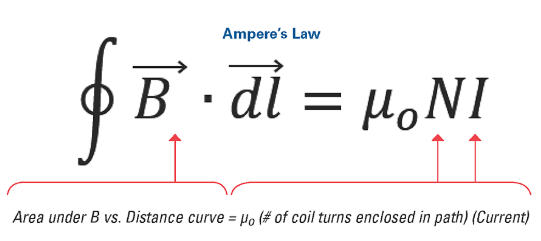# Ampere’s Law

Ampere’s Law and its Applications to Infinitely Long Straight Wire

Ampere’s law states that the magnetic field in the space around the electric current is proportional to an electric current that is serving as its source, just like the electric field in the space is proportional to the charge that is serving as its source. This law says that for any of the closed-loop path, a sum of length elements times in a magnetic field in a direction of length element that is equal to the permeability times, the electric current is enclosed in the loop.The full version of this law is one of the equations of the Maxwell, which describes the electromagnetic forces. Specifically, this law states that the created magnetic field by the electric current is proportional to the size of electric current with a specific constant of proportionality that is equal to the permeability of the free space.The field that is created by the long and straight wire is in the form of concentric circles. By moving further from the wire, the circles are getting further apart. In other words, it can be said that the field is getting weaker. Without the use of any calculus, an equation can be created for this law, and by using this equation, the strength of the field is easily calculated.

## Applications of Amperes Law to Infinitely Long Straight Wires

In some of the cases, the Ampere’s law can be used along with the symmetrical arguments for finding the unknown magnetic field. The magnetic fields have both magnitude and direction. By using the compasses, the direction of the magnetic field can be explored. The magnitude of the field can be determined by using the hall probe. The field that is present around the long and the straight wire exists in the circular loops. From this exploration, the right-hand tool 2 has emerged, and this rule is valid for the current segment, such as pointing of the thumb in the direction of current and the curling of fingers in the direction of magnetic field loops that are created by it.

Let suppose that there is a circle through the P, with the radius R and center O. At all the points, the magnetic induction of the circle will be the same and it will be in the tangential position to the circle, and that is also in the direction of the length element. So, by using the ampere’s law the magnetic induction can easily find out.